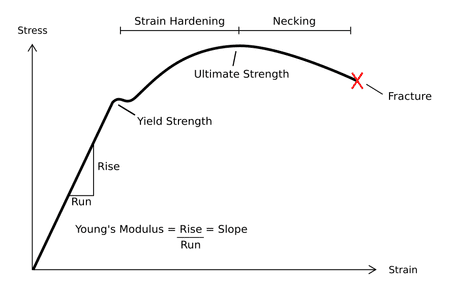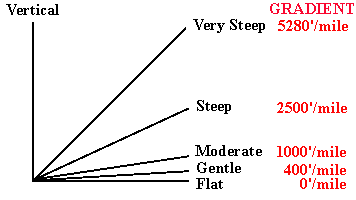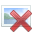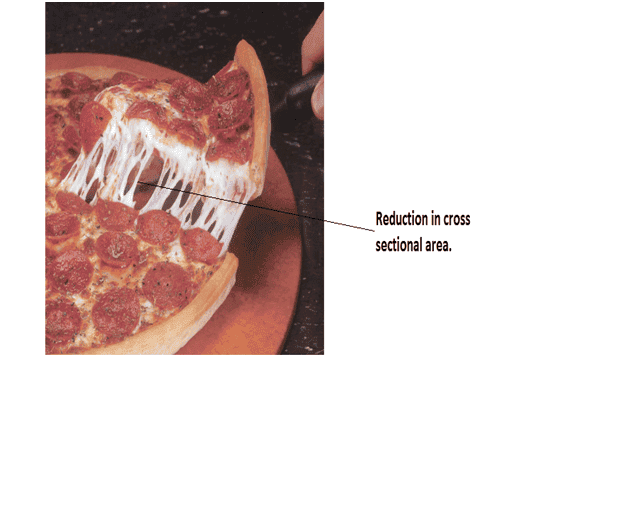# Stress vs strain curve

• gracy

#### gracy

How can we determine which curve has larger slope just by looking at curves.http://www.meritnation.com/img/shared/userimages/mn_images/image/a_2_12_2.png [Broken]
Which curve has larger slope?

Last edited by a moderator:

First, check to see that the axes are scaled the same, then look for which one is steeper.

Which curve has larger slope?
At what point?

which one is steeper.
That's what I wanted to ask how to determine which one is steeper?

how to determine which one is steeper
Without scales on the x and y axes, there is no way to determine slopes.

•gracy
Without scales on the x and y axes, there is no way to determine slopes.
I just have to tell which curve has larger slope,i.e I don't want to determine absolute or exact slope .I just want to compare slopes of the two curves which I have posted.Is it also not possible?

You can ASSUME both are scaled identically and pick which has the steeper initial slope (closer to vertical) --- but it's very poor form to make that sort of assumption, particularly between two entirely different graphic styles. It's likewise very poor graphic style to omit any suggestions of scale, but that takes ink, and ink costs publishers money.

•gracy
and pick which has the steeper initial slope
Curve 2 in my post,right?

Curve 2 in my post,right?
That's the one my eye tells me is steeper. I would still hate to wager large sums of money on what either of the plots actually represent numerically.

So steepness is determined by looking at how straight curve is?Or by how much closer the curve is with y axis?

I think the second one is correct.As this image depicts.Yes. You've taken calculus?

What is meant by set strain in stress vs strain curve?

You've taken calculus?
No.

set strain
"Set strain" is permanent deformation of an object. Under stress objects deform. Up to a certain limit, they deform elastically; that is, the deformation is NOT permanent, and if the stress is removed/relaxed, they will return to their original dimensions and shape. If stress is increased beyond that limit, when it is again relaxed or removed, the object will not return to its original shape and/or dimensions. Take a spring from a "dead" ball point pen refill, and stretch or compress it one cm. When you release it, it flies across the table/room, and when you finally find where it landed, it will be the original length. Stretch it to the length of your hand and release it, and you will notice that it's unable to recover it's original length of 3 to 4 cm.

As far as the calculus question, you will eventually be presented with the concept of "slope" which in x-y, or Cartesian, coordinates is defined as the limit as delta x goes to zero of delta y divided by delta x. It can be approximated by looking at a non-zero change in x and seeing how much y changes, and that's probably a more convenient way of looking at it for you for the moment.

•gracy
I understood what set strain is.It is strain which remains permanently .Last thing about strain vs stress I want to ask.What is neck in this type of curve?

Why stress decreases after point D in the curve below?When materials are actually pulled (just like taffy) apart to generate these curves, at some value of applied stress, the sample specimen (sometimes a square cross-section, sometimes a rectangular cross-section, sometimes round cross-section, and other cross section shapes) will stop "drawing" (elongating) uniformly over the length of the specimen, and at its weakest point will begin "necking," a local reduction of cross-sectional area that then has lower tensile strength than the rest of the specimen (lower area times tensile strength equals lower force it can resist) and this effect then leads to the final tensile failure.

•gracy
and at its weakest point will begin "necking,"
Can you please explain this further,Sir.

If you have a "fat" rubber band handy, look at its cross-section, or bungee cord for holding books together, and look at the "relaxed" cross-section. It's uniform over the length of whatever is (rubber band, bungee cord,...) As you stretch it, the cross-section decreases, again, uniformly over the length (for a given force, the cross-section is constant over the length) up to the point where it breaks. Trying to think of a material that will show "necking." Cheese on a pizza --- you pick up a slice, and the cheese sticks to the next slice, or the tray, and pulls ("draws") out into long thin strings? Occasionally you'll have a really thick blob of cheese that starts to draw, then just seems to "pull back into itself" at either end and leave the thin string connecting the two blobs? That's necking.

•gracyHave I interpreted correctly?

•Bystander
Yes.

But I am not getting one thing.Stress=force/area
If there is reduction in cross sectional area stress should increase than why stress decreases after point D (before breaking point)in my curve of post 17.

•Bystander
why stress decreases after point D
What's plotted is the total stress. It's not actually divided by the actual cross-sectional area of the "necked-down" section of the test specimen. This link
http://en.wikipedia.org/wiki/Ultimate_tensile_strength may be some help for you. Particularly the section showing the "red" vs. "blue" stress-strain curves, and the explanation that accompanies it describing the two cross-sectional areas used in plotting both of these curves, "A" and "A0."

http://en.wikipedia.org/wiki/Ultimate_tensile_strength may be some help for you.
Yes.It helped.Particularly this line
"when necking becomes substantial, it causes a reversal of the engineering stress–strain curve as the engineering stress is calculated assuming the original cross-sectional area before necking."
I have interpreted it (the curve of 17 post)in this way.Curve A to B obeys hooks law.Than B to C denotes plastic flow i.e strain increases without increase in stress as in your pizza analogy cheese starts to flow /pull then curve C to D depicts necking as cross sectional area decreases stress increases,lastly curve D to E indicates substantial increase in necking that's why we will consider original cross sectional area so stress will come down to original value|(less than necking region)that's why stress appears to decrease after point D in curve.
Correct?

You have got it.

•gracy
Thanks.You are such a nice teacher.God bless you.

Sir @Bystander I again want to start conversation with you,can I?

Sir @Bystander have you left the conversation with option ignore future messages?

But I am not getting one thing.Stress=force/area
If there is reduction in cross sectional area stress should increase than why stress decreases after point D (before breaking point)in my curve of post 17.

I also had problems wit this until I realized that the curves are obtained by forcing the extension of the sample with a very strong frame (i.e. Not by hanging a load on it). The force needed for that extension is then measured. If you try it the other way, the sample will just give way and break before reaching the interesting time.

Sir @Bystander I again want to start conversation with you,can I?
If the question is on-topic, so stress/strain relationship, just ask the question and either Bystander or somebody else will be able to help you. If the question is about something else, then please start another topic.

•gracy